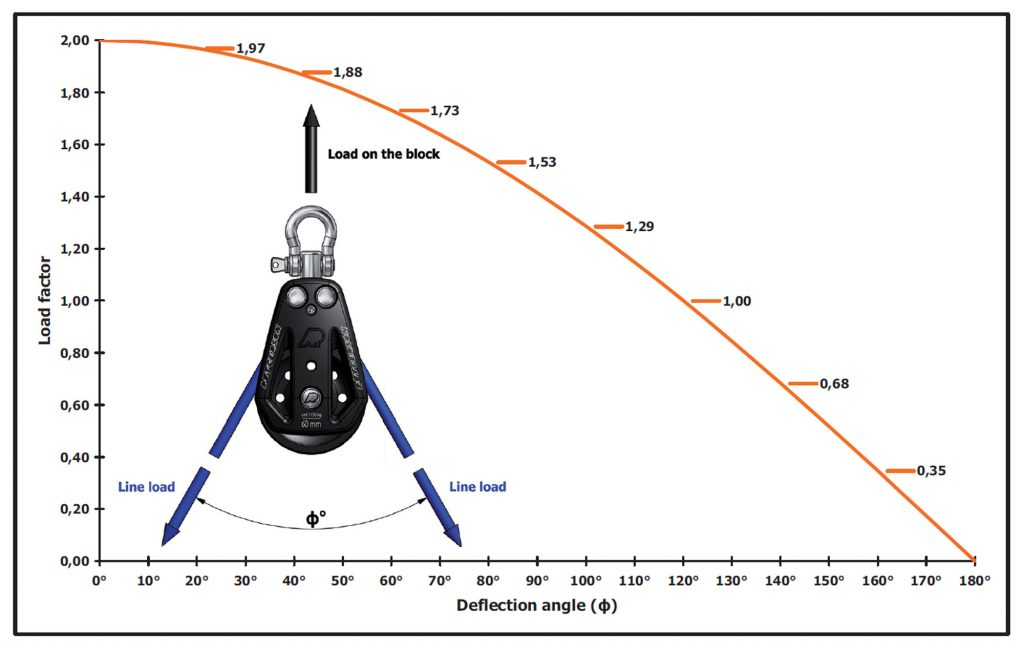Something we often overlook when we size a block is its installation.

In fact, after figuring out the load on the line, we need to check how this line passe through the block.

For a block used to lift your main sail, the haylard gos up and down forming a 0 degre angle. For a genoa traveller, the jibsheet goes throught with an open angle of ± 130 to 160 degres.Thus, the block load is determined by the angle and pulling force from the line. If the force is coming from the direct opposite side of the attachment point of the block, then the load factor will be 2X. So if you have 100 kg force in the lines and the angle Φ between them is 0° the block load will be 200 kg.

If the line force is 150 kg and the angle is 100° then the block load will be 194 kg (150kg * 1,29 = 193,4).Now all you have to do is to make sure you buy a block with a higher safe working load (SWL) than your calculation. The SWL is normally marked on the block. It can also be found in the product specification.